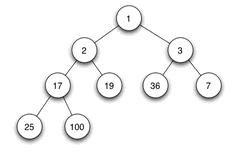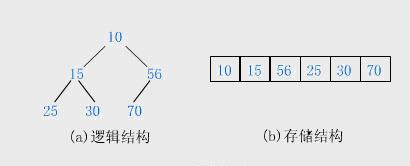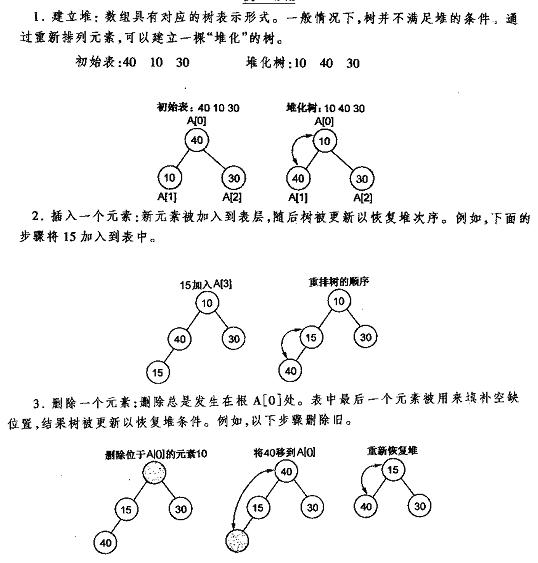• 粉丝 2
cleey

## 二叉堆的定义

1．父结点的键值总是大于或等于（小于或等于）任何一个子节点的键值。

2．每个结点的左子树和右子树都是一个二叉堆（都是最大堆或最小堆）。## 堆的存储## 堆的操作——插入删除### 堆的插入

```//  新加入i结点  其父结点为(i - 1) / 2
void MinHeapFixup(int a[], int i)
{
int j, temp;

temp = a[i];
j = (i - 1) / 2;      //父结点
while (j >= 0 && i != 0)
{
if (a[j] <= temp)
break;

a[i] = a[j];     //把较大的子结点往下移动,替换它的子结点
i = j;
j = (i - 1) / 2;
}
a[i] = temp;
}
```

```void MinHeapFixup(int a[], int i)
{
for (int j = (i - 1) / 2; (j >= 0 && i != 0)&& a[i] > a[j]; i = j, j = (i - 1) / 2)
Swap(a[i], a[j]);
}
```

```//在最小堆中加入新的数据nNum
void MinHeapAddNumber(int a[], int n, int nNum)
{
a[n] = nNum;
MinHeapFixup(a, n);
}
```

### 堆的删除

```//  从i节点开始调整,n为节点总数 从0开始计算 i节点的子节点为 2*i+1, 2*i+2
void MinHeapFixdown(int a[], int i, int n)
{
int j, temp;

temp = a[i];
j = 2 * i + 1;
while (j < n)
{
if (j + 1 < n && a[j + 1] < a[j]) //在左右孩子中找最小的
j++;

if (a[j] >= temp)
break;

a[i] = a[j];     //把较小的子结点往上移动,替换它的父结点
i = j;
j = 2 * i + 1;
}
a[i] = temp;
}
//在最小堆中删除数
void MinHeapDeleteNumber(int a[], int n)
{
Swap(a, a[n - 1]);
MinHeapFixdown(a, 0, n - 1);
}
```

## 堆化数组```//建立最小堆
void MakeMinHeap(int a[], int n)
{
for (int i = n / 2 - 1; i >= 0; i--)
MinHeapFixdown(a, i, n);
}
```

## 堆排序

```void MinheapsortTodescendarray(int a[], int n)
{
for (int i = n - 1; i >= 1; i--)
{
Swap(a[i], a);
MinHeapFixdown(a, 0, i);
}
}  ```